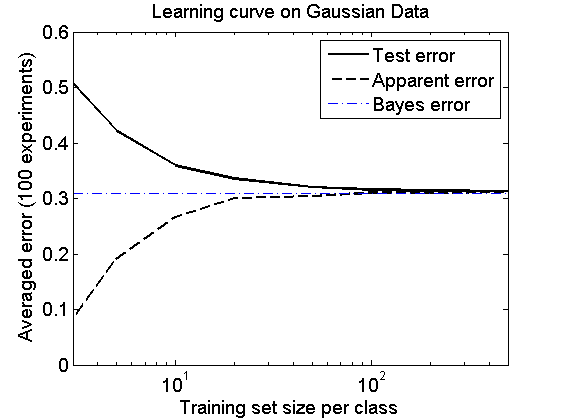# bayes_classifier

Example of the Bayes classifier, learning curves and the Bayes error

PRTools should be in the path.

Download the m-file from here. See http://37steps.com/prtools for more.

## Initialisation

delfigs
gridsize(300); % needed to show class borders accurately
randreset;     % take care of reproducability


## Define dataset based on 4 normal distributions

% Covariance matrices
G = {[1 0; 0 0.25],[0.02 0; 0 3],[1 0.9; 0.9 1],[3 0; 0 3]};
% Class means
U = [1 1; 2 1; 2.5 2.5; 2 2];
% Class priors
P = [0.2 0.1 0.2 0.5];


Note that the the first three classes have small prior probabilities, and the probability of the background class is much larger.

## Show maximum densities (not the sum!!) (standard Matlab)

This part of the code may be skipped. It is only needed for generating the 3D density plot.

% Domain of interest
x1 = -2:.1:6; x2 = -1.5:.1:5.5;
% Make a grid
[X1,X2] = meshgrid(x1,x2);
% Compute maximum density times prior probs for the 4 classes
F = zeros(numel(x2)*numel(x1),1);
for n=1:numel(G)
F = max(F,P(n)*mvnpdf([X1(:) X2(:)],U(n,:),G{n}));
end
% Create plot
F = reshape(F,length(x2),length(x1));
surf(x1,x2,F);
caxis([min(F(:))-.5*range(F(:)),max(F(:))]);
axis([min(x1) max(x1) min(x2) max(x2) 0 max(F(:))])
xlabel('x1'); ylabel('x2'); zlabel('Maximum Probability Density');
title('3D view of the weighted densities.')
fontsize(14);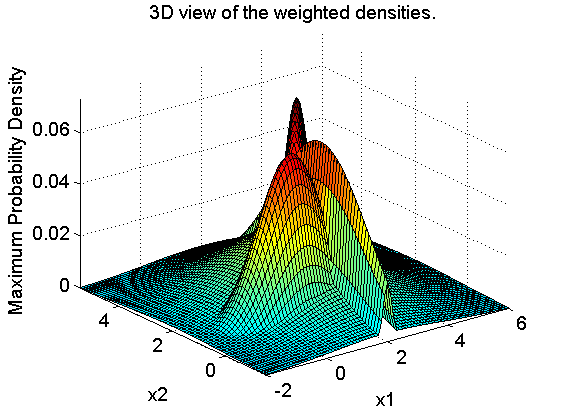The 3D plot shows for every point of the grid the maxima of the four density functions, each multiplied by their class priors.

## Compute Bayes classifier by PRTools

U = prdataset(U,[1:numel(G)]'); % create labeled dataset for class means
U = setprior(U,P);              % add class priors
G = cat(3,G{:});                % put covariance matrices in 3D array
w = nbayesc(U,G);               % Bayes classifier


## Show class domains according to Bayes classifier

figure;
axis equal
axis([min(x1) max(x1) min(x2) max(x2)]);
plotc(w,'col');
box on;
xlabel('Feature 1');
ylabel('Feature 2');
title('Domains assigned to the four classes by the Bayes classifier')
fontsize(14);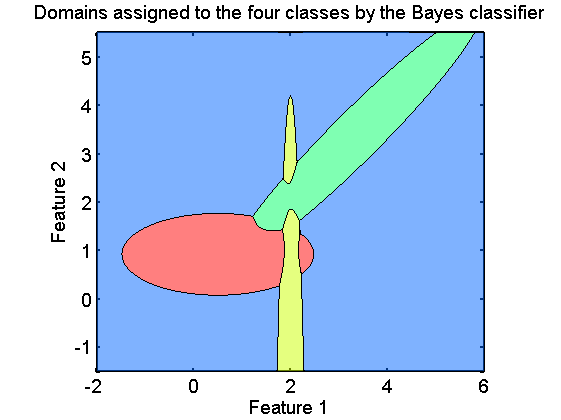## Show classifiers in prior weighted density plot

figure;
axis equal
axis([min(x1) max(x1) min(x2) max(x2)]);
plotm(w); % plot weighted density map
plotc(w); % show classifier boundaries
box on
title('Weighted density plots and the Bayes classifier')
fontsize(14);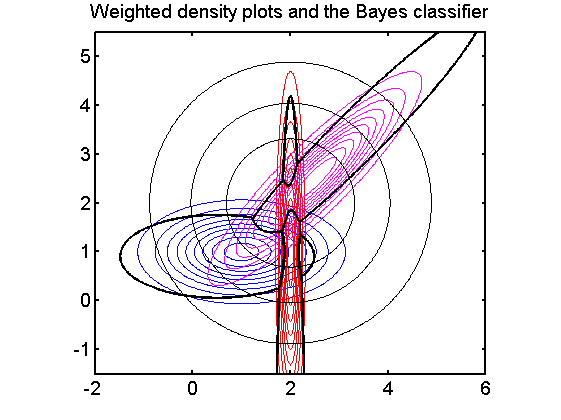Note that the classifier passes exactly the points of equal densities of the classes.

## Computation of the Bayes error

If the distributions are known the Bayes error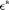can be computed by integrating the areas of overlap. Here this is done be a Monte Carlo procedure: a very large dataset of a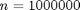objects per class, is generated from the true class distributions and tested on the Bayes classifier. The standard deviation of this estimate is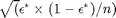n = 1000000;
a = gendatgauss(n*ones(1,4),U,G);
f = a*w*testc;
s = sqrt(f*(1-f)/n);
fprintf('The estimated Bayes error is %6.4f with standard deviation %6.4f\n',f,s);

The estimated Bayes error is 0.3098 with standard deviation 0.0005


## The learning curve

Here it is shown how the expected classification error approximates the Bayes error for large training set sizes. It is eeseential that a classifier is used that can model the true class distributions. In this case this is fulfilled as the true distributions are normal and the classifier, qdc, can model them.

figure;
a = gendatgauss(10000*ones(1,4),U,G); % generate 10000 objects per class
e = cleval(a,qdc,[3 5 10 20 50 100 200 500],100);
plote(e);
hold on;
feval(e.plot,[min(e.xvalues) max(e.xvalues)],[f f],'b-.');
legend off
legend('Test error','Apparent error','Bayes error')
fontsize(14);